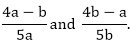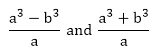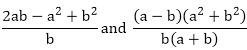# RD Sharma Class 10 Ex 3.4 Solutions Chapter 3 Pair of Linear Equations in Two Variables

In this chapter, we provide RD Sharma Class 10 Ex 3.4 Solutions Chapter 3 Pair of Linear Equations in Two Variables for English medium students, Which will very helpful for every student in their exams. Students can download the latest RD Sharma Class 10 Ex 3.4 Solutions Chapter 3 Pair of Linear Equations in Two Variables pdf, Now you will get step by step solution to each question.

# Chapter 3: Pair of Linear Equations in Two Variables Exercise – 3.4

### Question: 1

Solve the system of equations:

x + 2y + 1 = 0 and 2x – 3y – 12 = 0

### Solution:

x + 2y + 1 = 0 …. (i)

2x – 3y – 12 = 0 …….. (ii)

Here a= 1, b= 2, c= 1

a= 2, b= -3, c= – 12

By cross multiplication method,

Now,

x = 3

And,

= y = – 2

The solution of the given system of equation is 3 and – 2 respectively.

### Question: 2

Solve the system of equations:

3x + 2y + 25 = 0, 2x + y + 10 = 0

### Solution:

3x + 2y + 25 = 0 …. (i)

2x + y + 10 = 0 ….. (ii)

Here a= 3, b= 2, c= 25

a= 2, b= 1, c= 10

By cross multiplication method,

Now,

x = 5

And,

y = – 20

The solution of the given system of equation is 5 and -20 respectively.

### Question: 3

Solve the system of equations:

2x + y = 35, 3x + 4y = 65

### Solution:

2x + y = 35 …. (i)

3x + 4y = 65 ….. (ii)

Here a= 2, b= 1, c= 35

a= 3, b= 4, c= 65

By cross multiplication method,

Now,

x = 15

And,

y = 5

The solution of the given system of equation is 15 and 5 respectively.

### Question: 4

Solve the system of equations:

2x – y – 6 = 0, x – y – 2 = 0

### Solution:

2x – y = 6 …. (i)

x – y = 2 ….. (ii)

Here a= 2, b= -1, c= 6

2= 1, b= -1, c= 2

By cross multiplication method,

Now,

x = 4

And,

y = 2

The solution of the given system of equation is 4 and 2 respectively

### Question: 5

Solve the system of equations:

### Solution:

Taking 1/x = u

Taking 1/y = v

u + v = 2 …… (iii)

u – v = 6 ….  (iv)

By cross multiplication method,

Now,

u/4 = 1/-2

u = – 2

And,

– v/ – 8 = 1/ – 2

v = – 4

1/u = x

X = – 1/2

y = –1/4

The solution of the given system of equation is -1/2 and -1/4 respectively.

### Question: 6

Solve the system of equations:

ax + by = a – b, bx – ay = a + b

### Solution:

ax + by = a – b …. (i)

bx – ay = a + b ….. (ii)

Here a= a, b= b, c= a – b

a= b, b= -a, c= a + b

By cross multiplication method,

Now,

x = 1

And,

y = – 1

The solution of the given system of equation is 1 and -1 respectively.

### Question: 7

Solve the system of equations:

x + ay – b = 0, ax – by – c = 0

### Solution:

x + ay – b = 0 ….. (i)

ax – by – c = 0 ……. (ii)

Here a= 1, b= a, c= – b

a= a, b= – b , c= – c

By cross multiplication method,

Now,

And,

The solution of the given system of equation isrespectively.

### Question: 8

Solve the system of equations:

ax + by = a2

bx + ay = b2

### Solution:

ax + by = a…. (i)

bx + ay = b….. (ii)

Here a= a, b= b, c= a2

a= b, b= a, c= b2

By cross multiplication method,

Now,

And,

The solution of the given system of equation isrespectively.

### Question: 9

Solve the system of equations:

### Solution:

The given system of equations are:

5u – 2v = -1

15u + 7v = -10

Here a= 5, b= – 2, c= 1

a= 15, b= 7, c= 10

By cross multiplication method,

Now,

x + y = 5 …. (i)

And,

v = 1

x – y =  1 …… (ii)

2x = 6

x = 3

Putting the value of x in equation (i)

3 + y = 5

y = 2

The solution of the given system of equation is 3 and 2 respectively.

### Question: 10

Solve the system of equations:

### Solution:

Let 1/x = u

Let 1/y = v

The given system of equations becomes:

2u + 3v = 13 …… (i)

5u – 4v = – 2 …. (ii)

By cross multiplication method,

Now,

u = 2

And,

v = 3

The solutions of the given system of equations are 1/2 and 1/3 respectively.

### Question: 11

Solve the system of equations:

### Solution:

The given system of equations are:

57u + 6v = 5

38u + 21v = 9

Here a= 57, b= 6, c= – 5

a= 38, b= 21, c= – 9

By cross multiplication method,

Now,

x + y = 19 ….. (i)

And,

x – y = 3 … (ii)

2x = 22

x = 11

Putting the value of x in equation (i)

11 + y = 19

y = 8

The solution of the given system of equation is 11 and 8 respectively.

### Question: 12

Solve the system of equations:

### Solution:

a= a, b= – b, c= b– a2

By cross multiplication method

x = a

y = b

Hence the solution of the given system of equation are a and b respectively.

### Solution:

By cross multiplication method

x = a2

y = b2

The solution of the given system of equation are a2  and brespectively.

ax + by = a+ b2

### Solution:

Here, a= a, b= b, Let c= – (a+ b2)

By cross multiplication method

x = a

y = b

The solution of the given system of equations are a and b respectively.

### Question: 15

2ax + 3by = a + 2b

3ax + 2by = 2a + b

### Solution:

The given system of equation is

2ax + 3by = a + 2b …… (i)

3ax + 2by = 2a + b ….. (ii)

Here a= 2a, b= 3b, c= – (a + 2b)

a= 3a, b= 2b, c= – (2a + b)

By cross multiplication method

Now,

The solutions of the system of equations arerespectively.

5ax + 6by = 28

3ax + 4by = 18

### Solution:

The systems of equations are:

5ax + 6by = 28 …. (i)

3ax + 4by = 18  …. (ii)

Here a= 5a, b= 6b, c= – (28)

a= 3a, b= 4b, c= – (18)

By cross multiplication method

Now,

The solution of the given system of equation is 2/a and 3/b.

### Question: 17

(a + 2b)x + (2a – b)y = 2

(a – 2b)x + (2a + b)y = 3

### Solution:

The given system of equations are:

(a + 2b)x + (2a – b)y = 2 ……. (i)

(a – 2b)x + (2a + b)y = 3 ….. (ii)

Here a= a + 2b, b= 2a – b, c= – (2)

a= a – 2b, b= 2a + b, c= – (3)

By cross multiplication method:

The solution of the system of equations are

### Question: 18

Solve the system of equations:

### Solution:

The given systems of equations are:

From equation (i)

From equation (ii)

x + y – 2a= 0

a= 1, b= 1, c= – 2a2

By cross multiplication method:

The solutions of the given system of equations arerespectively.

### Question: 19

Solve the system of equations:

### Solution:

The system of equation is given by:

bx + cy = a + b ……. (i)

From equation (i)

bx + cy – (a + b) = 0

From equation (ii)

2abx – 2acy – 2a(a – b) = 0 …. (iv)

By cross multiplication

x = a/b

And,

y = b/c

The solution of the system of equations are a/b and b/c.

### Question: 20

Solve the system of equations:

(a – b)x +(a + b)y = 2a– 2b2(a + b)(x + y) = 4ab

### Solution:

The given system of equations are:

(a – b)x + (a + b)y = 2a– 2b2  ….. (i)

(a + b)(x + y) = 4ab      …… (ii)

From equation (i)

(a – b)x + (a + b)y – 2a– 2b= 0

= (a – b)x + (a + b)y – 2(a– b2) = 0

From equation (ii)

(a – b)x + (a – b)y – 4ab = 0

Here, a= a – b, b= a + b, c= – 2(a+ b2)

Here, a= a + b, b= a + b, c= – 4ab

By cross multiplication method

Now,

The solution of the system of equations arerespectively.

### Question: 21

Solve the system of equations:

a2x + b2y = c2

b2x + a2y = d2

### Solution:

The given system of equations are:

a2x + b2y = c….. (i)

b2x + a2y = d…… (ii)

Here, a= a2, b= b2, c= – c2

Here, a= b2, b= a2, c= – d2

By cross multiplication method

Now,

The solution of the given system of equations arerespectively.

### Question: 22

Solve the system of equations:

2(ax – by + a + 4b = 0

2(bx + ay) + b – 4a = 0

### Solution:

The given system of equation may be written as:

2(ax – by + a + 4b = 0 ….. (i)

2(bx + ay) + b – 4a = 0 …. (ii)

Here, a= 2a, b= -2b, c= a + 4b

Here, a= 2b, b= 2a, c= b – 4a

By cross multiplication method

y = 2

The solution of the given pair of equations are -1/2 and 2 respectively.

### Question: 23

Solve the system of equations:

6(ax + by) = 3a + 2b

6(bx – ay) = 3b – 2a

### Solution:

The systems of equations are

6(ax + by) = 3a + 2b …… (i)

6(bx – ay) = 3b – 2a ……. (ii)

From equation (i)

6ax + 6by – (3a + 2b) = 0  …… (iii)

From equation (ii)

6bx – 6ay – (3b – 2a) = 0 …… (iv)

Here, a= 6a, b= 6b, c= – (3a + 2b)

Here, a= 6b, b= -6a, c= – (3b – 2a)

By cross multiplication method

The solution of the given pair of equations are 1/2 and 1/3 respectively.

### Question: 24

Solve the system of equations:

### Solution:

The given systems of equations are

Taking 1/x = u

Taking 1/y = v

The pair of equations becomes:

a2u – b2v = 0

a2bu + b2av – (a + b) = 0

Here, a= a2, b= -b2, c= 0

Here, a= a2b, b= b2a, c= – (a + b)

By cross multiplication method

The solution of the given pair of equations are 1/a2 and 1/b2 respectively.

### Question: 25

Solve the system of equations:

mx – my = m+ n2

x + y = 2m

### Solution:

mx – my = m+ n…… (i)

x + y = 2m …….. (ii)

Here, a= m, b= -n, c= – (m+ n2)

Here, a= 1, b= 1, c= – (2m)

By cross multiplication method

x = m + n

y = m – n

The solutions of the given pair of equations are m+n and m-n respectively.

### Question: 26

Solve the system of equations:

ax – by = 2ab

### Solution:

The given pair of equations are:

ax – by = 2ab ….. (ii)

Here, a= a, b= – b, c= – (2ab)

By cross multiplication method

x = b

y = – a

The solution of the given pair of equations are b and – a respectively.

### Question: 27

Solve the system of equations:

x + y – 2ab = 0 ……. (ii)

### Solution:

x + y – 2ab = 0 ……. (ii)

By cross multiplication method

x = ab

y = ab

The solutions of the given pair of equations are ab and ab respectively.

All Chapter RD Sharma Solutions For Class10 Maths

I think you got complete solutions for this chapter. If You have any queries regarding this chapter, please comment on the below section our subject teacher will answer you. We tried our best to give complete solutions so you got good marks in your exam.